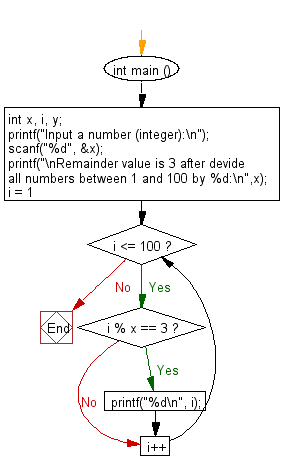﻿ C : Divide 1 to 100 by 3 and find the remainder

# C Exercises: Divide all numbers between 1 and 100 and find all those numbers where remainder value is 3

## C Basic Declarations and Expressions: Exercise-111 with Solution

Write a C program that accepts an integer from the user and divides all numbers between 1 and 100. Print those numbers where the remainder value is 3.

Sample Solution:

C Code:

``````#include <stdio.h>

int main () {
// Declare variables
int x, i, y;

// Prompt user for input
printf("Input a number (integer):\n");

// Read an integer from user and store it in 'x'
scanf("%d", &x);

// Print a message about the remainder condition
printf("\nRemainder value is 3 after divide all numbers between 1 and 100 by %d:\n", x);

// Loop to check for remainder condition
for (i = 1; i <= 100; i++){
if (i % x == 3){
printf("%d\n", i);
}
}

return 0; // End of program
}
``````

Sample Output:

```Input a number (integer):
65

Remainder value is 3 after divide all numbers between 1 and 100 by 65:
3
68
```

Flowchart:C programming Code Editor:

What is the difficulty level of this exercise?

Test your Programming skills with w3resource's quiz.

﻿